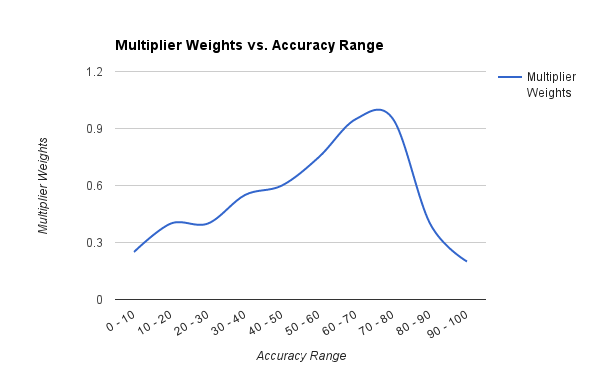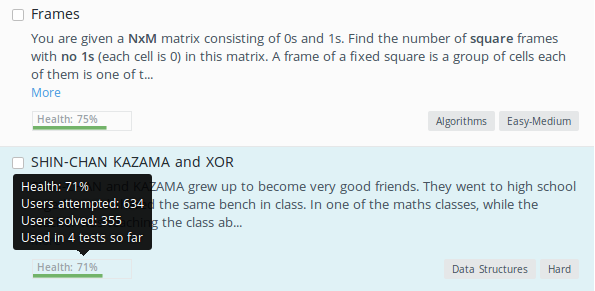## HackerEarth Question Library: Stats, Usage Analysis and Health

31 Oct 2015

We, at HackerEarth, cater a huge number of questions in Assessment tool. Recruiters can choose from wide varieties of Multiple Choice and Programming/Coding questions to assess candidates. Every week or two, new questions are added to the Questions Library. As the time passed, thousands of questions got stacked up and recruiters started to have a hard time figuring out what questions to choose from such a huge library.

We, developers, work closely with our sales team to understand recruiters’ needs. Sometimes we directly get in touch with recruiters to provide on call technical support and understand how they use the product and what improvements can be made to the product in order to make it more easier to use. That’s how we figured it was about time we helped recruiters figure the best questions out of our library and hence we released a feature called “Health”.

### What it means?

Health, in context of any library question, indicates how usable the question is. It is not only about question’s difficulty level. We consider various factors such as number of users attempted the question, users solved, times the question has been used and when was the question last used while determining Health of any question. Simple and short, higher the health, more usable the question.

### How we calculate the Health value?

The whole process was split into 3 parts:

1. Data Segregation and Analysis
2. Data Structure to store Health data
3. Mathematical formula to calculate Health value

#### 1. Data Segregation and Analysis

We tried to gather as much data as possible for any question. Then, filter out the ones that could help us in finding the health value.

• Number of tests in which the question was used
• When was the question last used in any test
• Question accuracy (Number of users who solved it correctly / Number of users attempted)
• Problem ratings (Ratings submitted by user for any question)
• Problem tags
• When was the question added
• How frequently the question is used
• When was the question last used

We figured that high weighing factors in the Health of any questions were question accuracy, number of times the question has been used and when was the question last used. We stuck to only 3 factors to keep things easy and started to build a basic version of question health.

#### 2. Data Structure to store Health data

Each question can be used in many tests. Every time we load question in library, we will have to calculate health of each of them by analyzing their use in all those tests. So, writing a brute forcer is probably not a good idea considering the number of database hits it would make.

Our requirement is that it should be possible to get health data of a list of questions in single query. This calls for a simple generic `Health` model that goes somewhat like this:

This `Health` model is generic, meaning that it can be used for any object of any model. Next, we need a helper model using which we can populate `Health` model anytime we want. The helper model will be specific to objects of different models. Such structure would help us in future, in case we want to extend Health feature.

For questions health, we create a helper model `ProblemUsageData` which stores question analytics, based on the factors we discussed earlier. We update this model as and when a question is used in any test. Later, to update `Health` model we have to formulate a tiny mathematical equation using the attributes of `ProblemUsageData` model.

That’s all with the data structure, let’s move on to Health calculation.

#### 3. Mathematical formula to calculate Health value

The three factors that we consider while generating health can be obtained through `ProblemUsageData` model as follows:

First comes `accuracy`, which as simple as this:

Next, we have `times_used`, to which we multiply some constant which can be found through following graph. Pick the range according to question `accuracy` and look for the respective multiplier. This graph was generated after a lot of hit and trial and it will be different for different types of question such as Multiple Choice, Programming/Coding etc.Lastly, from `last_used_event` field of `ProblemUsageData` we get when was the question last used. We have some threshold cooldown number of days until a question is again safely reusable.

Finally, we directly calculate health percentage by following formula

The multiplying factors `0.65`, `0.15` and `0.20` were again determined through hit and trial on known data sets. We make a check that none of these factors exceed 100.

Now that we are done with all the calculation, we can show data using one simple query which looks like this:

Final data at UI level looks like this, on hover we show some stats about the question:Got a burning question you want to get answered?, ask it in the comments or mail me at ravi[at]hackerearth[dot]com.

Posted by Ravi Ojha.